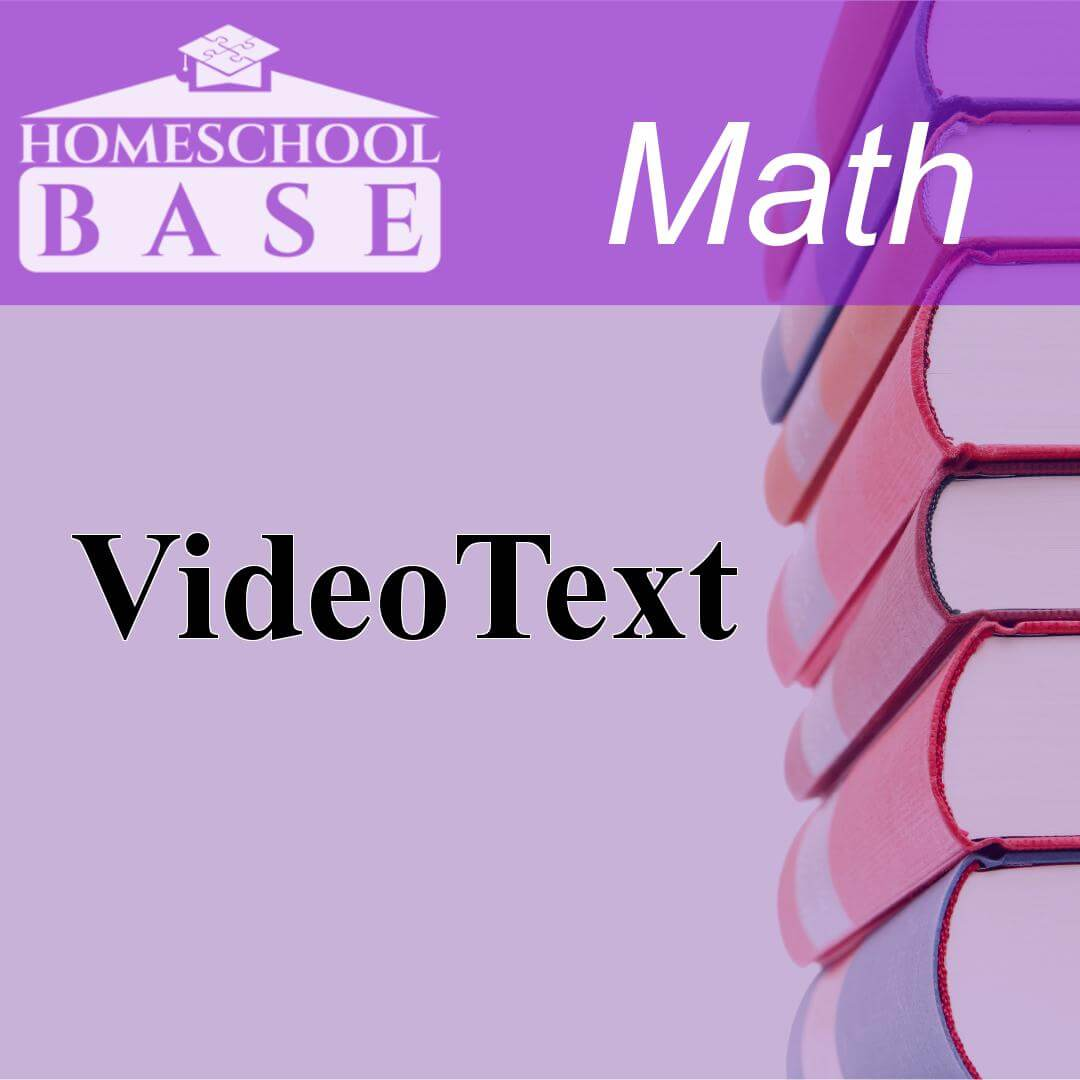« Back to curriculum

Let's find the best math curriculum for your 11th grader! This page has a filtered collection of the most popular and commonly used homeschool curriculum that can be used for 11th grade math.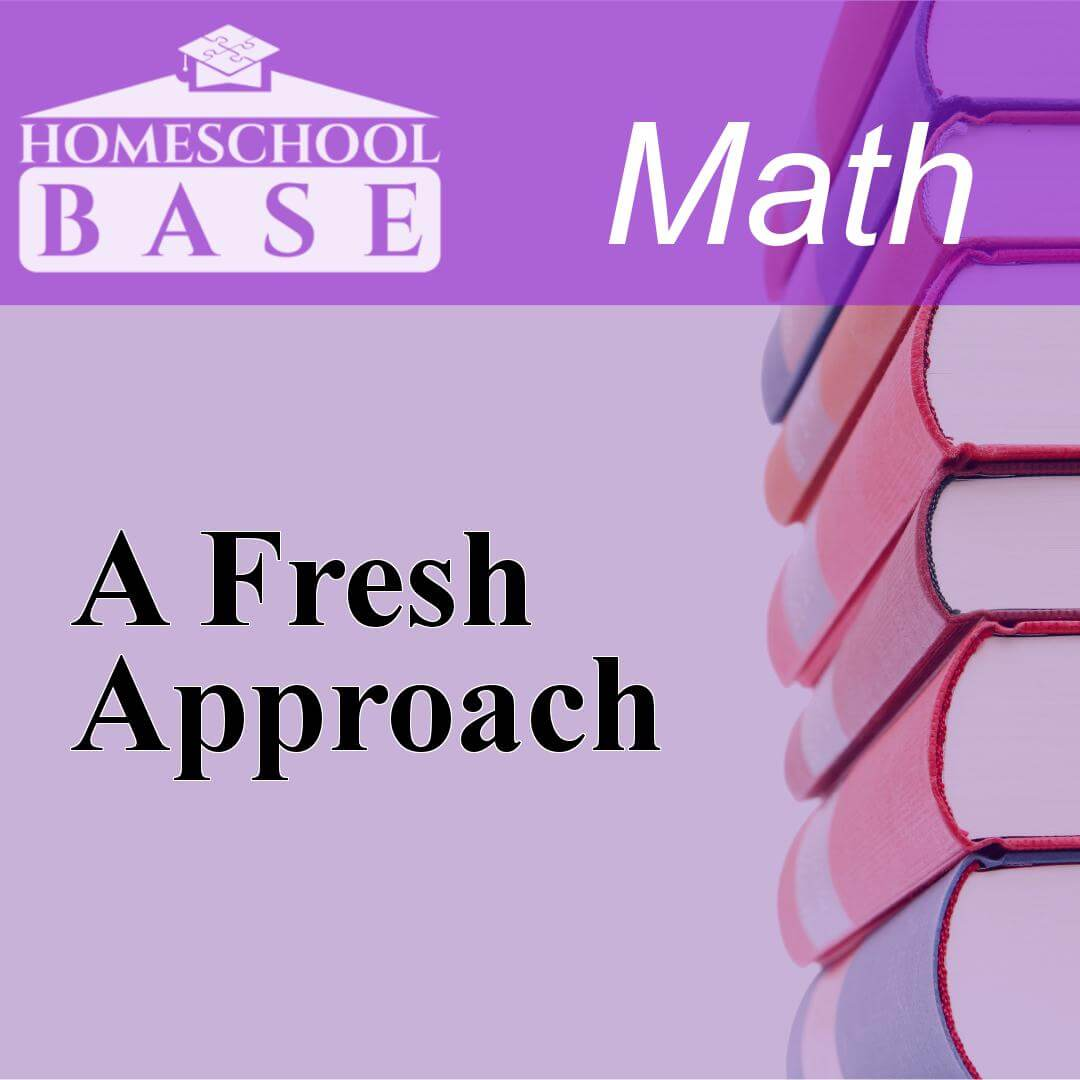## A Fresh Approach

A Fresh Approach is a Math curriculum for grades 8-12. This program teaches by using the sequential method. The average amount of teacher involvement on the parent(s) is: low. This curriculum does not...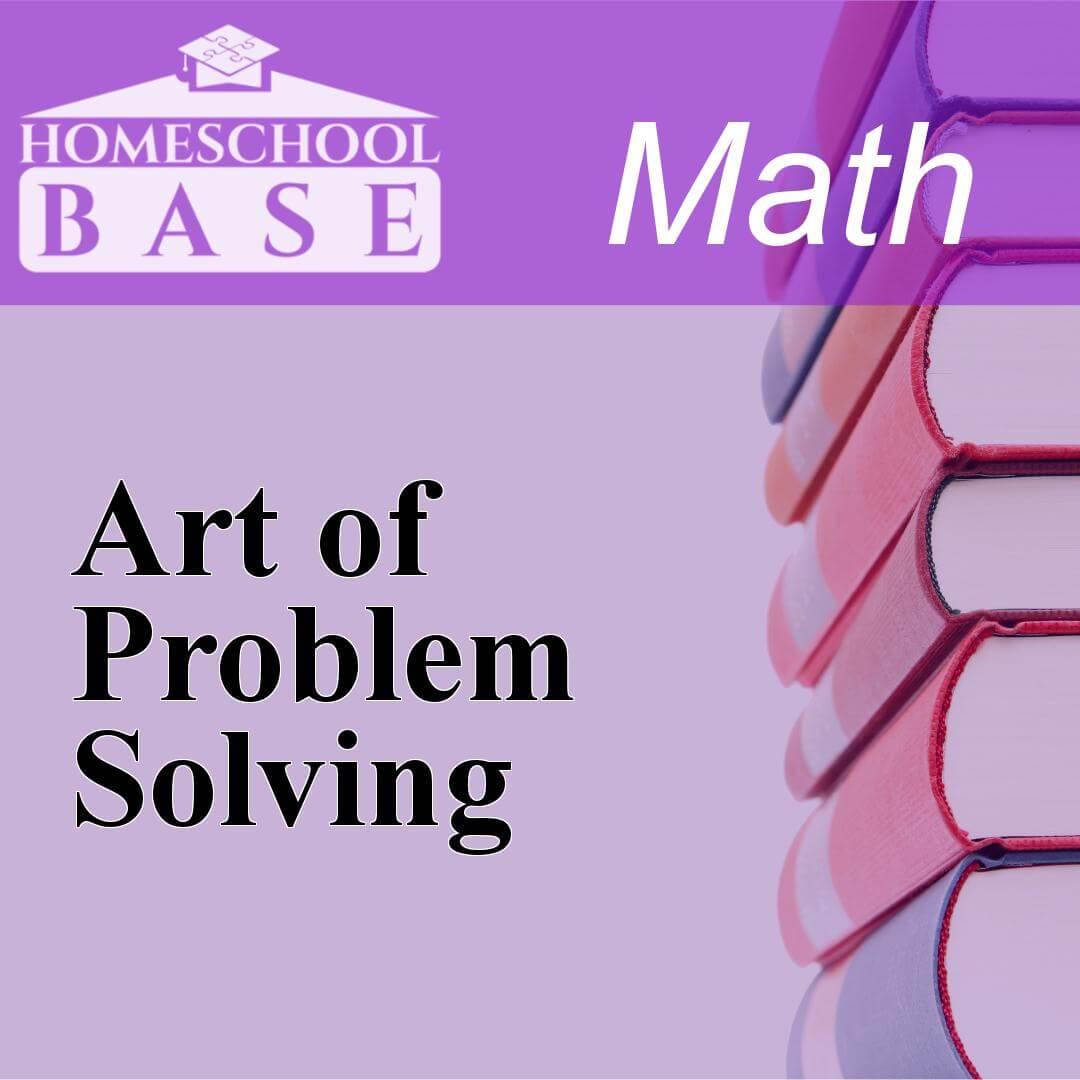## Art of Problem Solving

Art of Problem Solving is a Math curriculum for grades 6-12. This program teaches by using the sequential method. The average amount of teacher involvement on the parent(s) is: low. This curriculum d...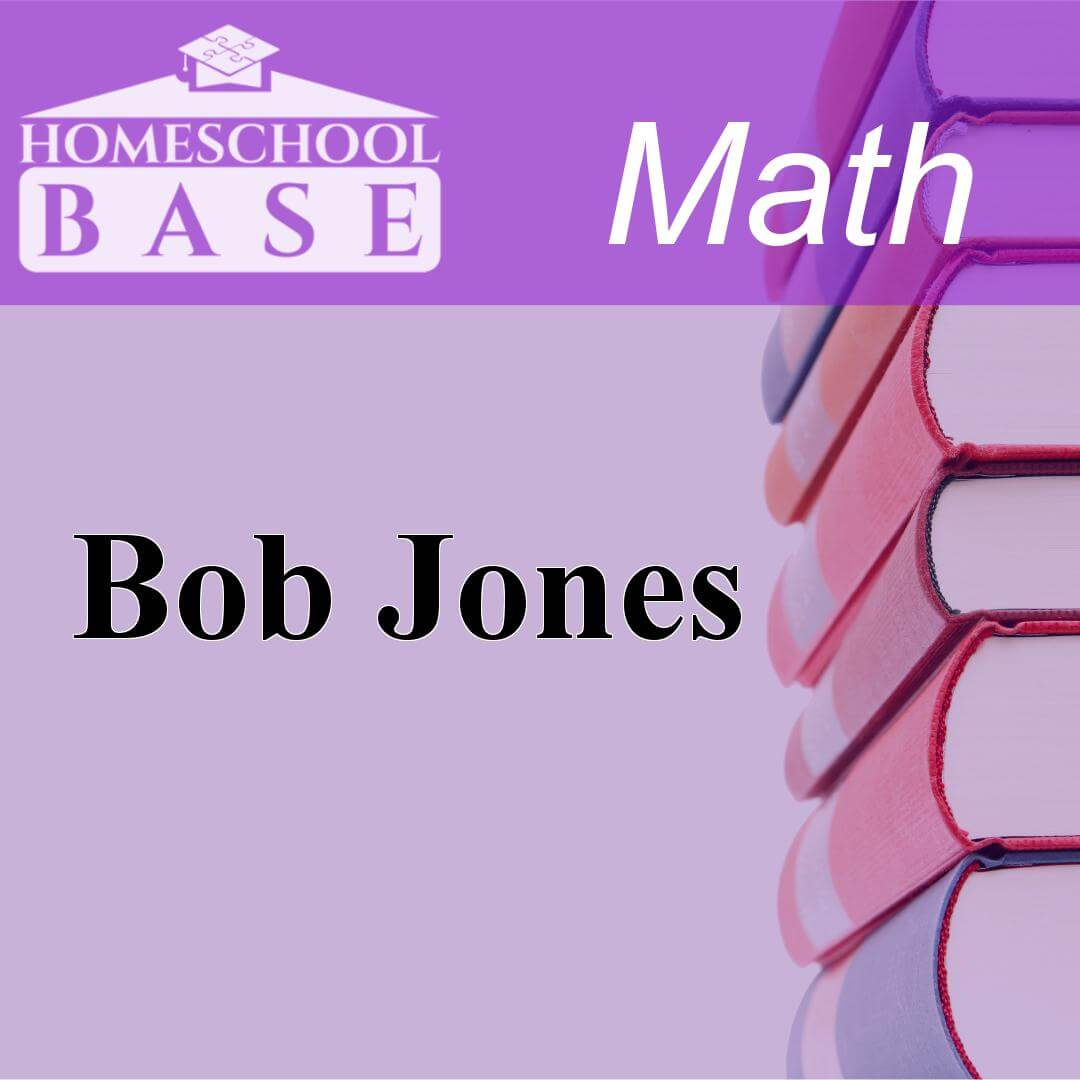## Bob Jones

Bob Jones is a Math curriculum for grades K-12. This program teaches by using the spiral method. The average amount of teacher involvement on the parent(s) is: high. This curriculum does not conform/a...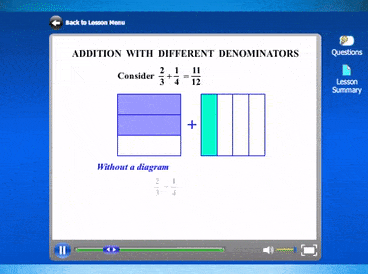## CTC Math Curriculum

CTC Math gives homeschoolers access to all lessons across all grade levels. Levels span from Kindergarten to Trigonometry. CTC Math provides a full mathematics curriculum for K-8. After 8th grade, CTC...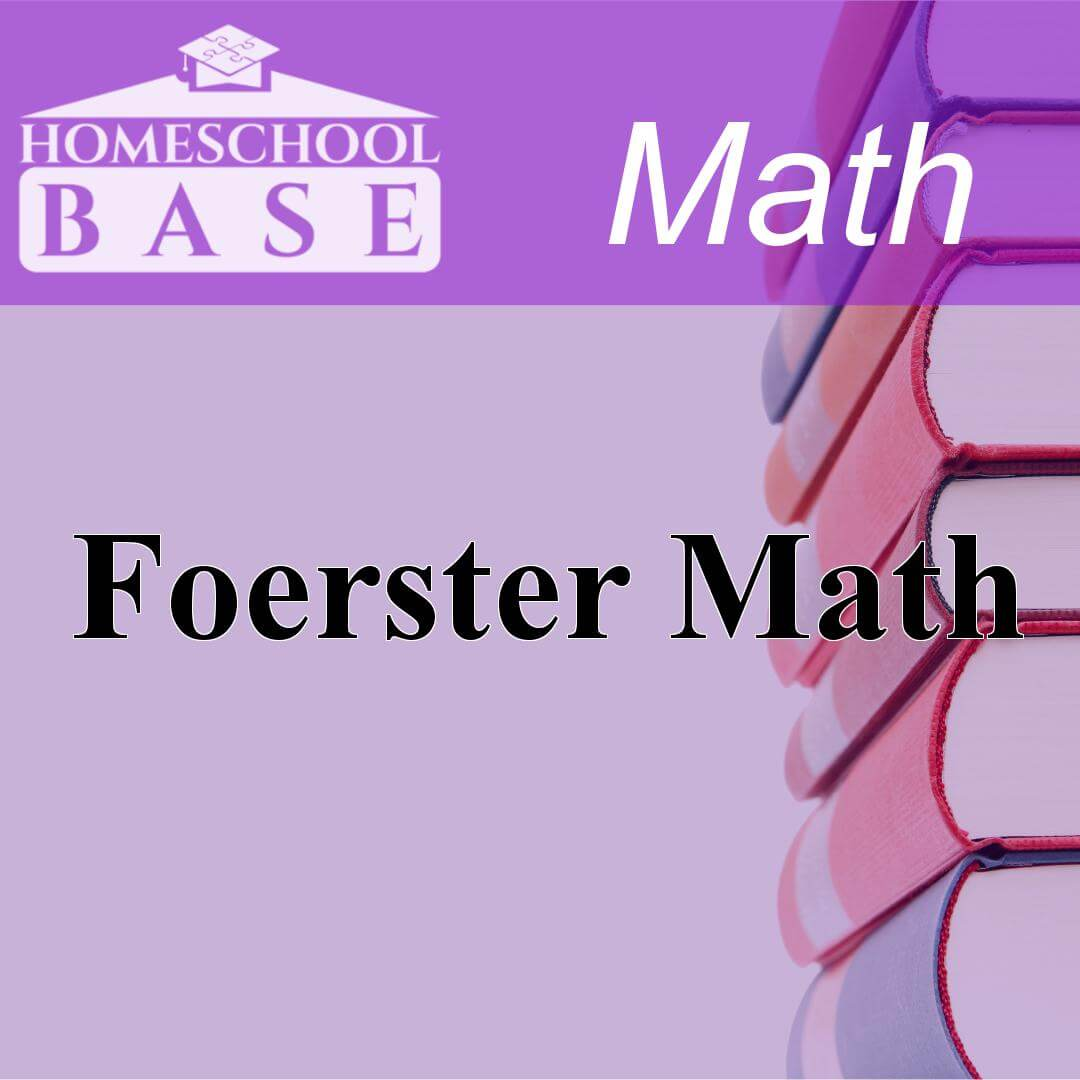## Foerster Math

Foerster Math is a Math curriculum for grades 9-12. This program teaches by using the sequential method. The average amount of teacher involvement on the parent(s) is: low. This curriculum does not co...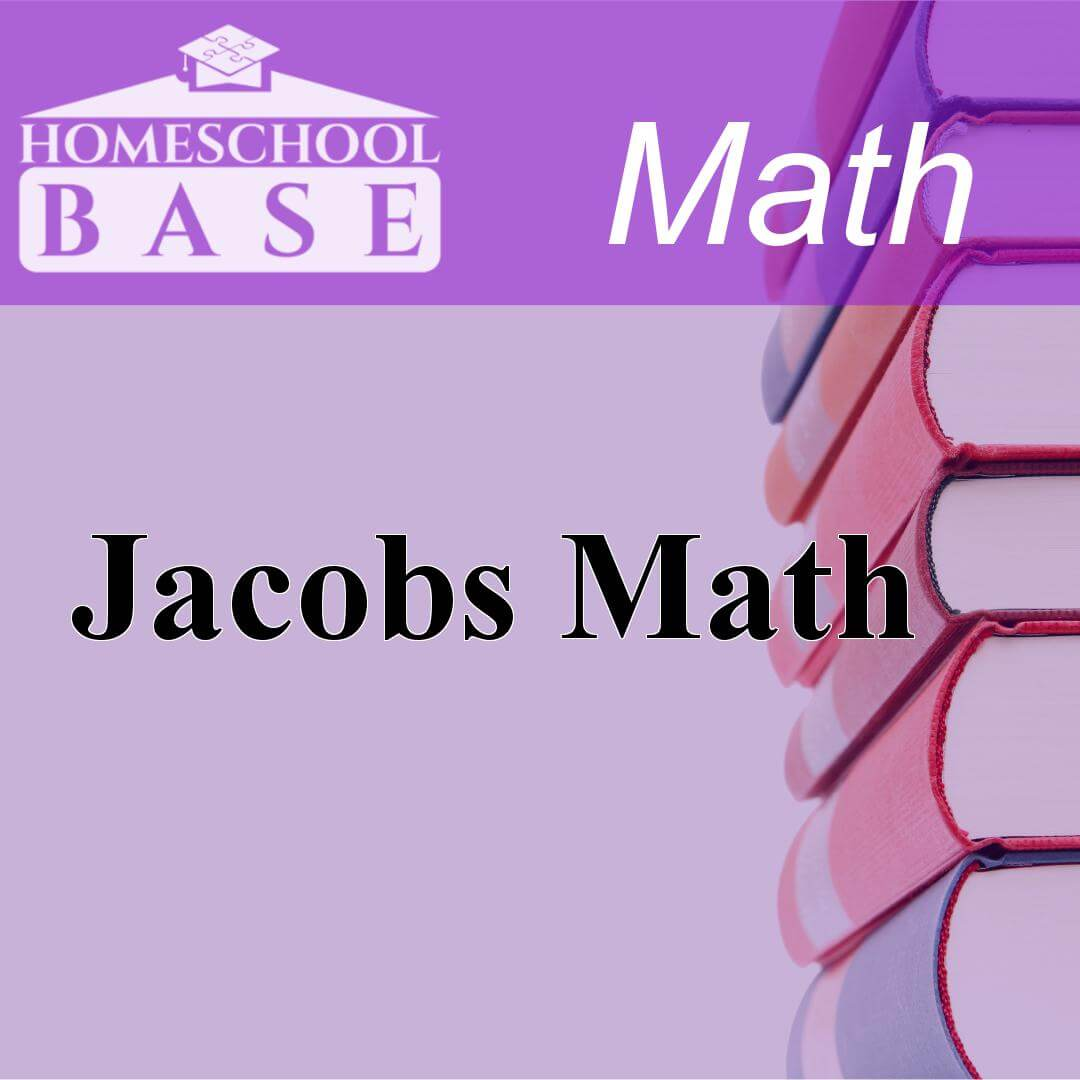## Jacobs Math

Jacobs Math is a Math curriculum for grades 9-12. This program teaches by using the sequential method. The average amount of teacher involvement on the parent(s) is: low. This curriculum does not conf...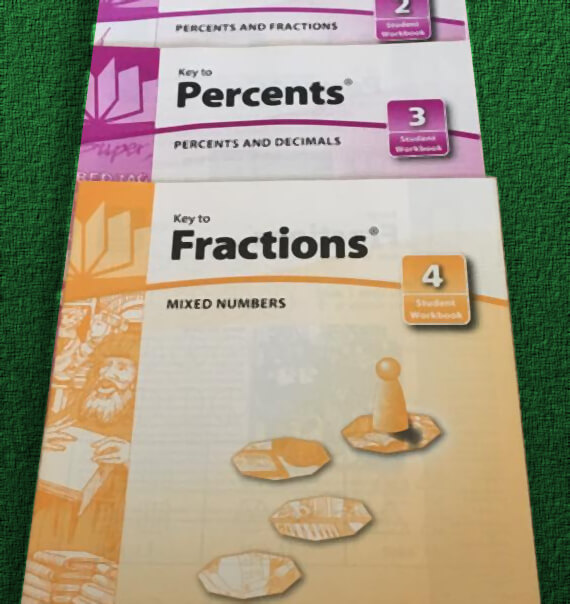## Key Curriculum Press

Key Curriculum Press publishes math curriculum like the Key to series and Miquon. Key Curriculum Press was acquired by McGraw-Hill Education in 2012. Key Curriculum Press developed inquiry-based mathe...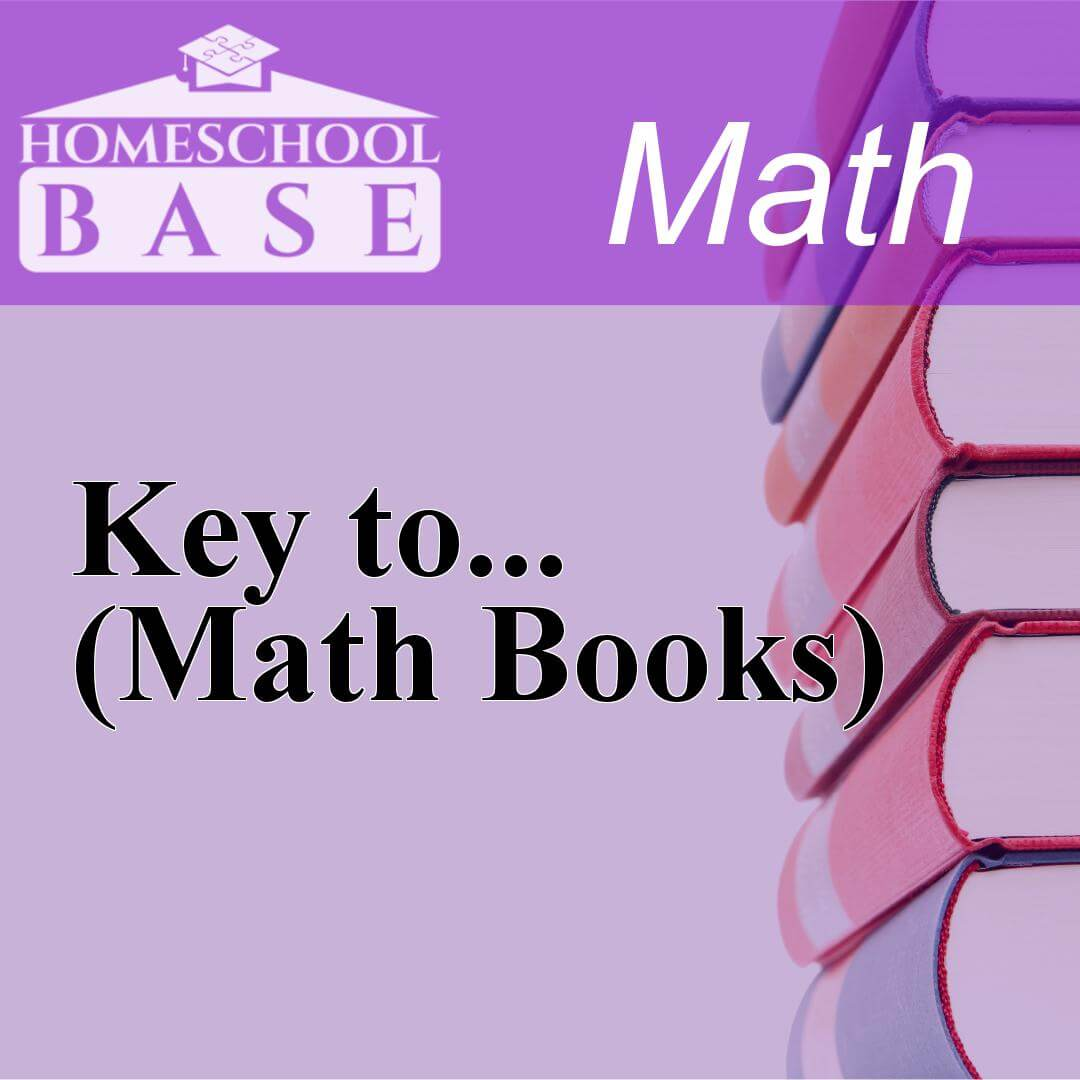## Key to... (Math Books)

Key to... (Math Books) is a Math curriculum for grades 4-12. This program teaches by using the conceptual-topical method. The average amount of teacher involvement on the parent(s) is: medium. This cu...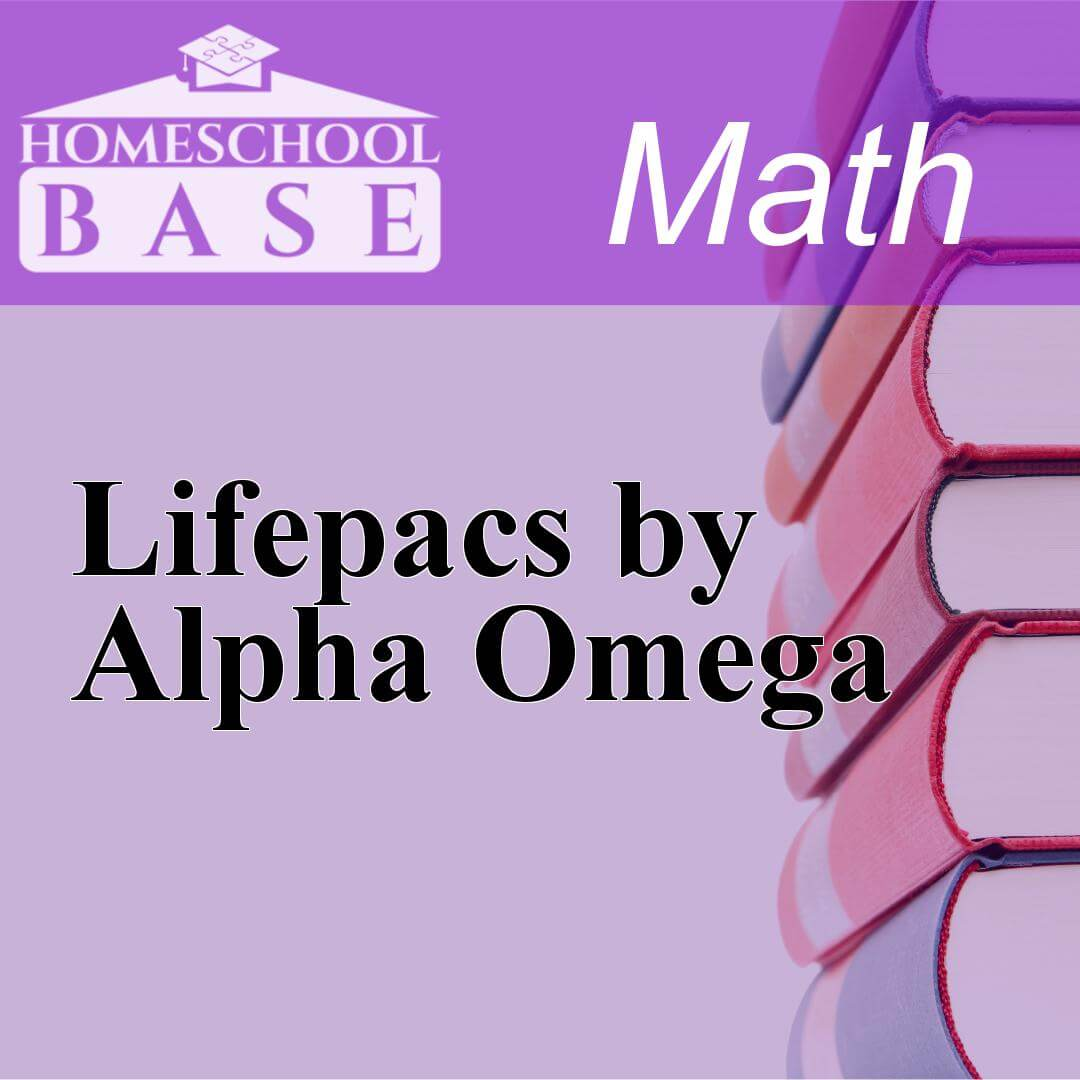## Lifepacs by Alpha Omega

Lifepacs by Alpha Omega is a Math curriculum for grades K-12. This program teaches by using the sequential method. The average amount of teacher involvement on the parent(s) is: medium. This curriculu...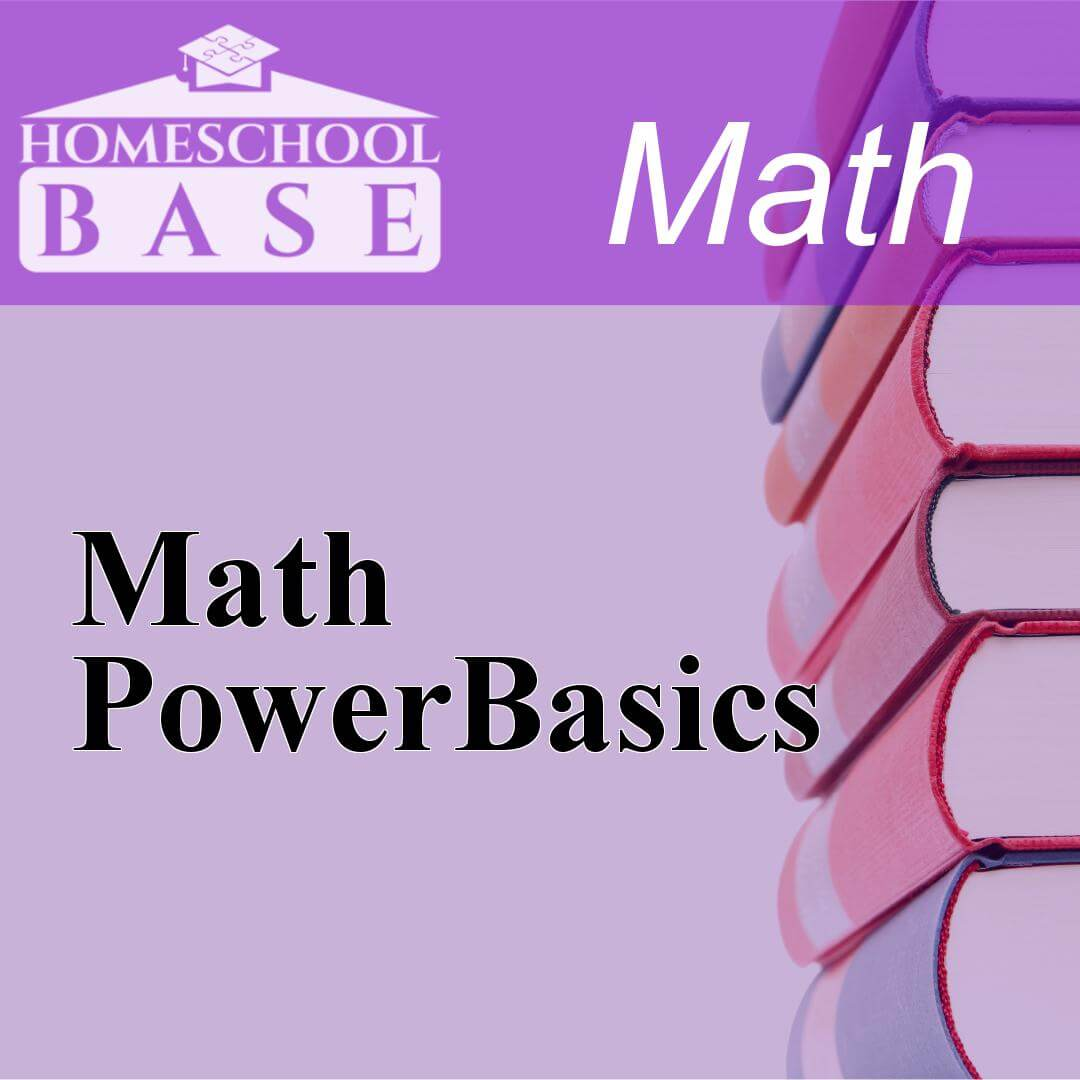## Math PowerBasics

Math PowerBasics is a Math curriculum for grades 6-12. This program teaches by using the sequential method. The average amount of teacher involvement on the parent(s) is: low. This curriculum does not...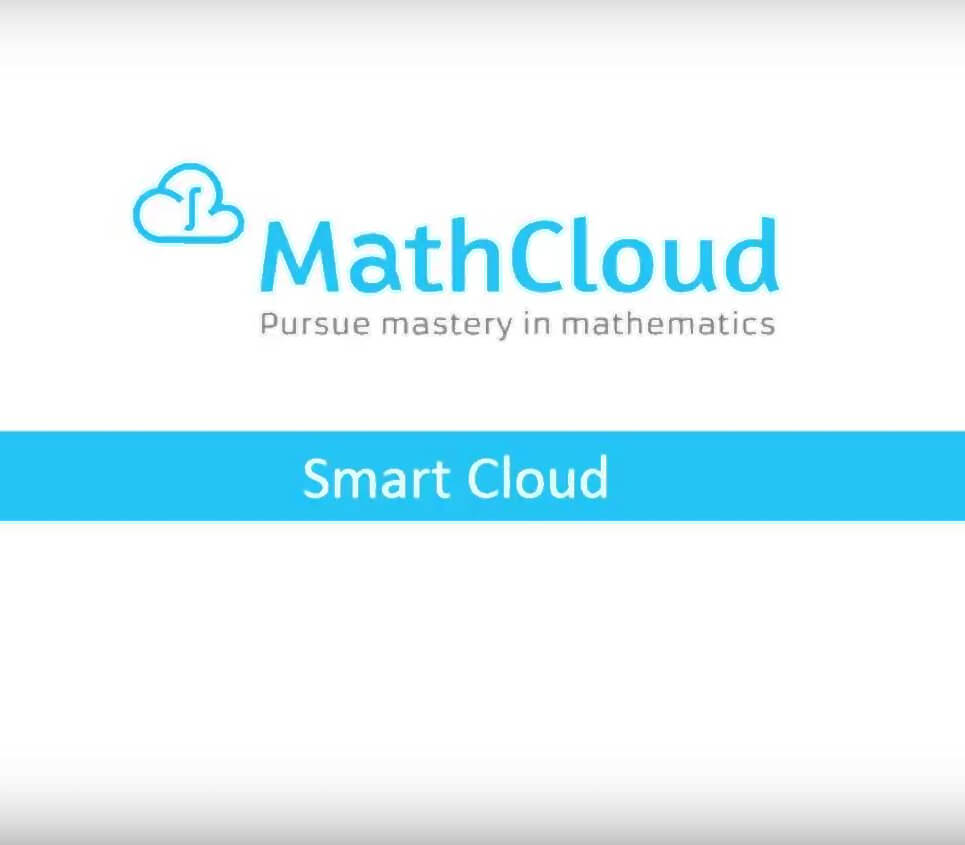## MathCloud

MathCloud offers a new approach to learning mathematics. Rather than directing students to memorize solutions and techniques, MathCloud teaches students to break complex problems down into parts and u...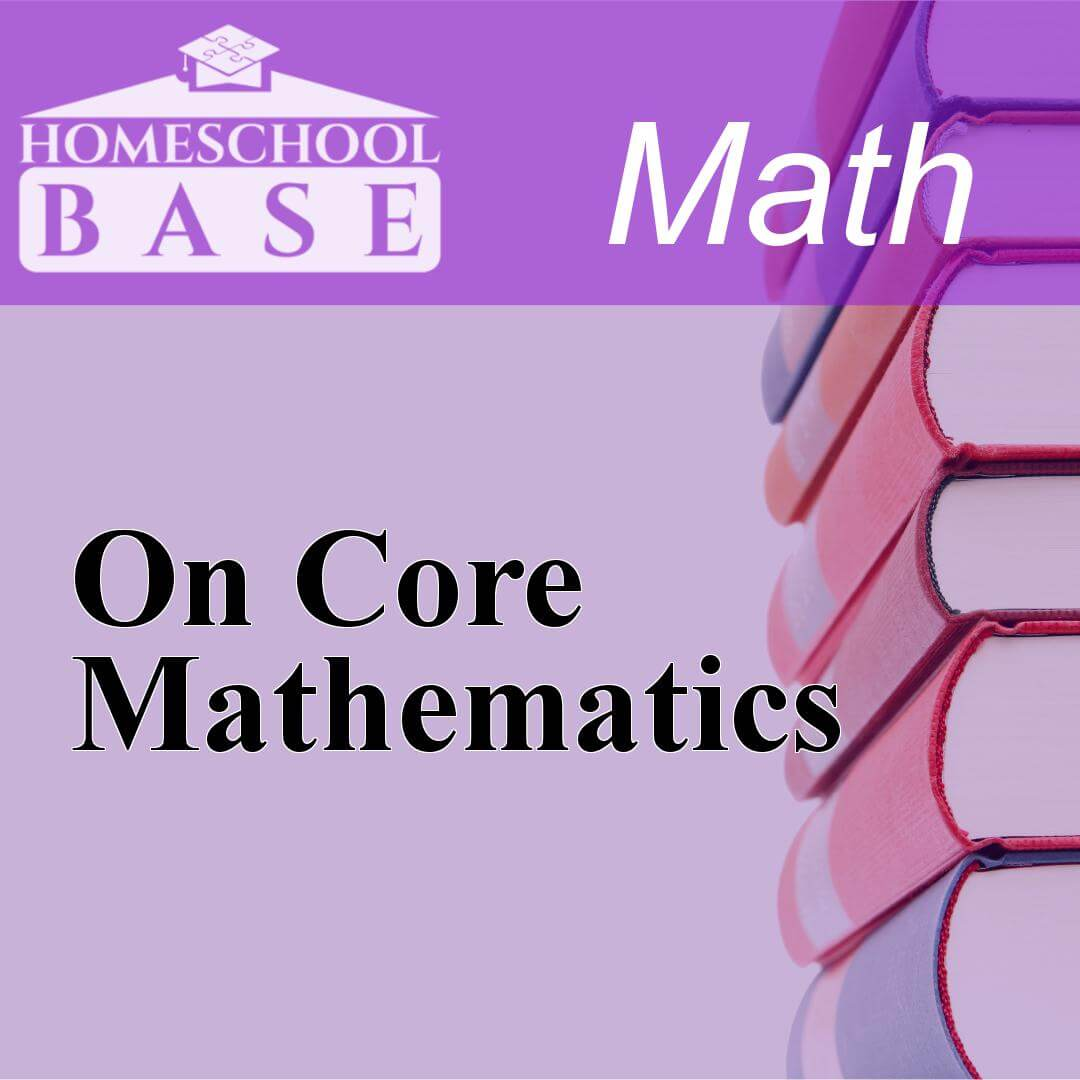## On Core Mathematics

On Core Mathematics is a Math curriculum for grades K-12. This program teaches by using the conceptual-topical method. The average amount of teacher involvement on the parent(s) is: medium. This curri...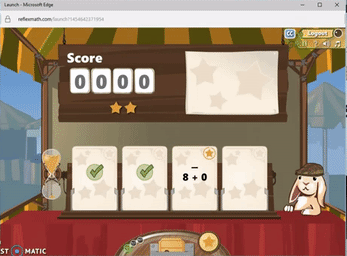## Reflex Math

Read our review of the best online math drill program and save 44% on a subscription! Reflex Math publishes high quality games that children really do enjoy playing. Reflex Math does a surprisingly go...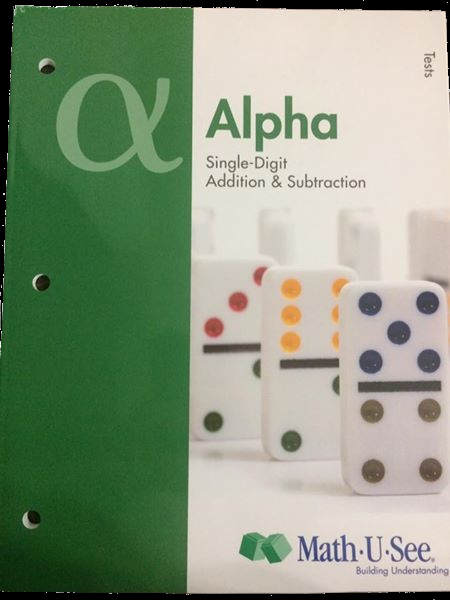## Math-U-See: Complete K-12 Math

Designed by a homeschool dad, Math-U-See is a manipulative-based math curriculum for K-12 that was specifically created with homeschoolers and small groups in mind. Math-U-See utilizes video instructi...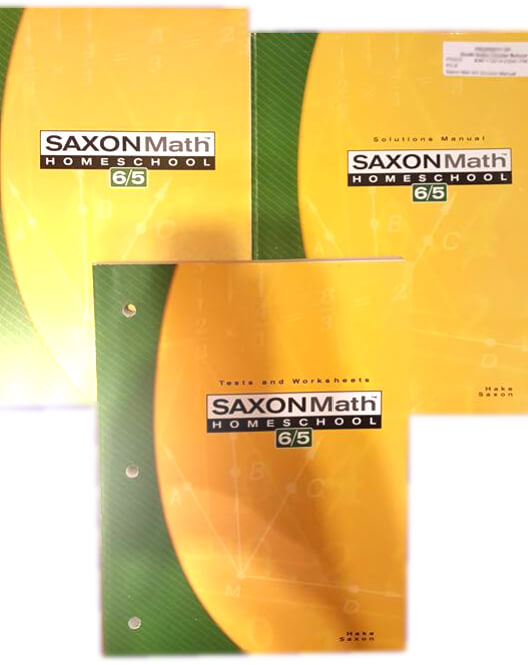## Saxon Math

Saxon Math Homeschool is one of the nation's most comprehensive and well-researched math programs. Extremely popular among homeschool families, Saxon Math boasts more than 30 years of success for grad...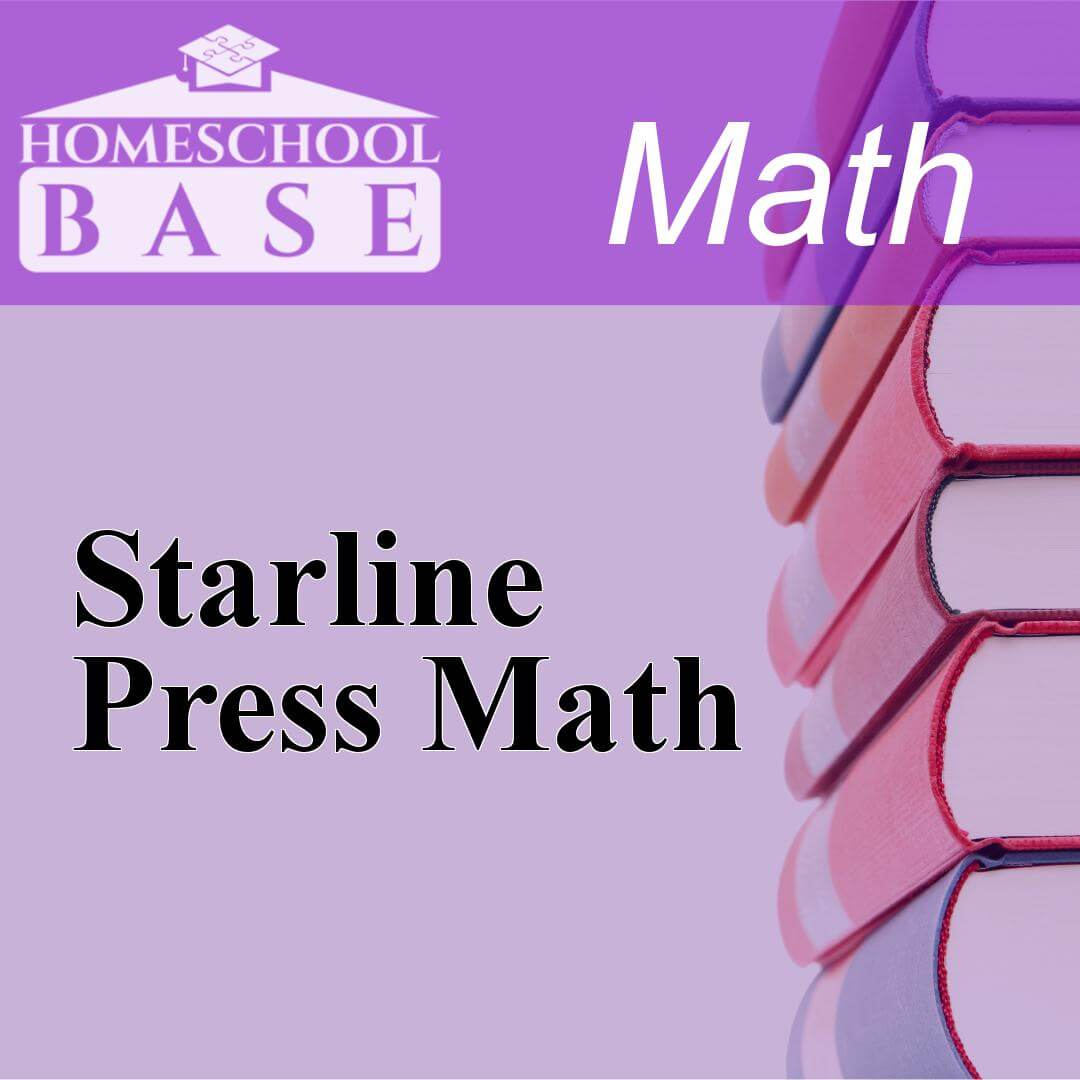## Starline Press Math

Starline Press Math is a Math curriculum for grades 3-12. This program teaches by using the sequential method. The average amount of teacher involvement on the parent(s) is: low. This curriculum does ...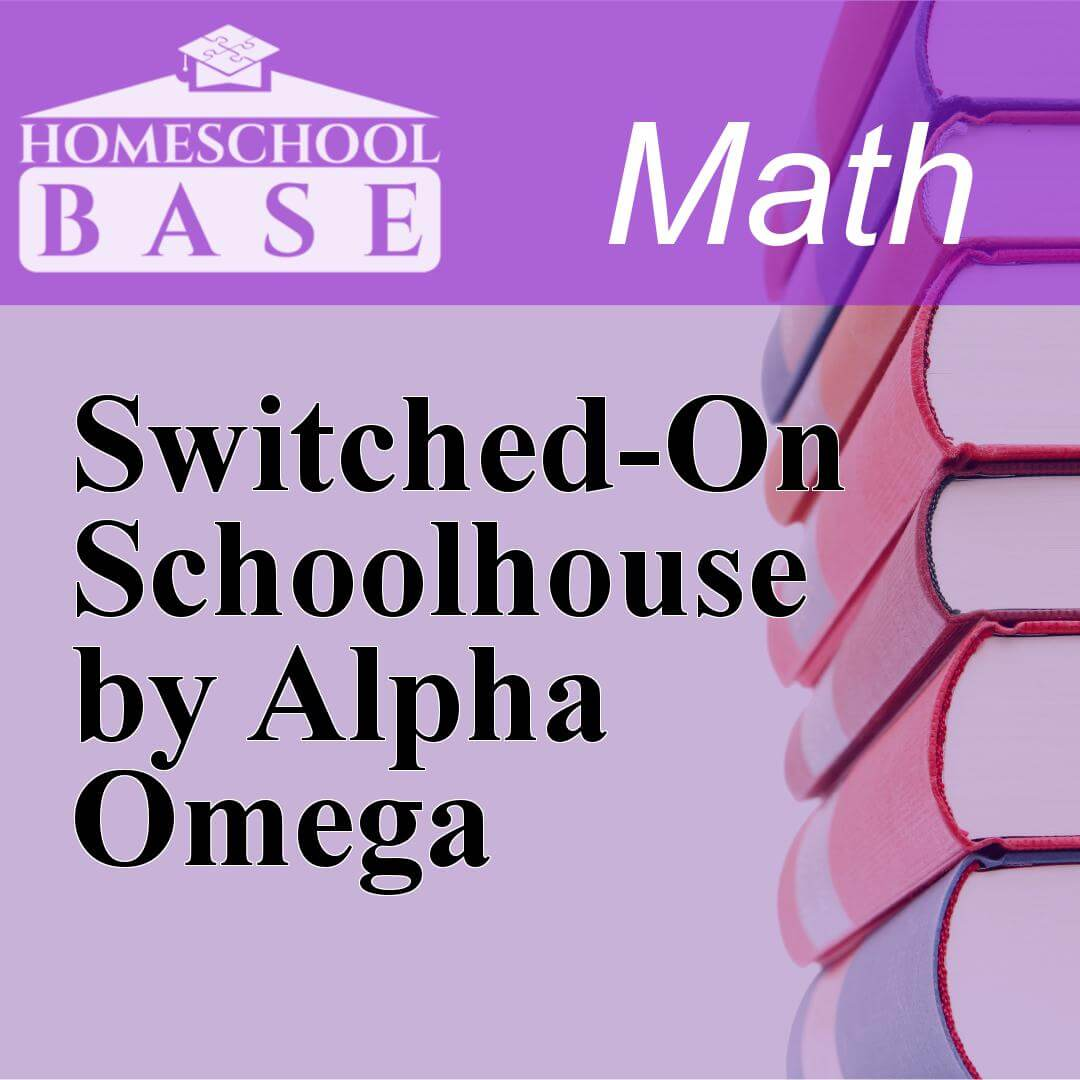## Switched-On Schoolhouse by Alpha Omega

Switched-On Schoolhouse by Alpha Omega is a Math curriculum for grades 3-12. This program teaches by using the sequential method. The average amount of teacher involvement on the parent(s) is: low. Th...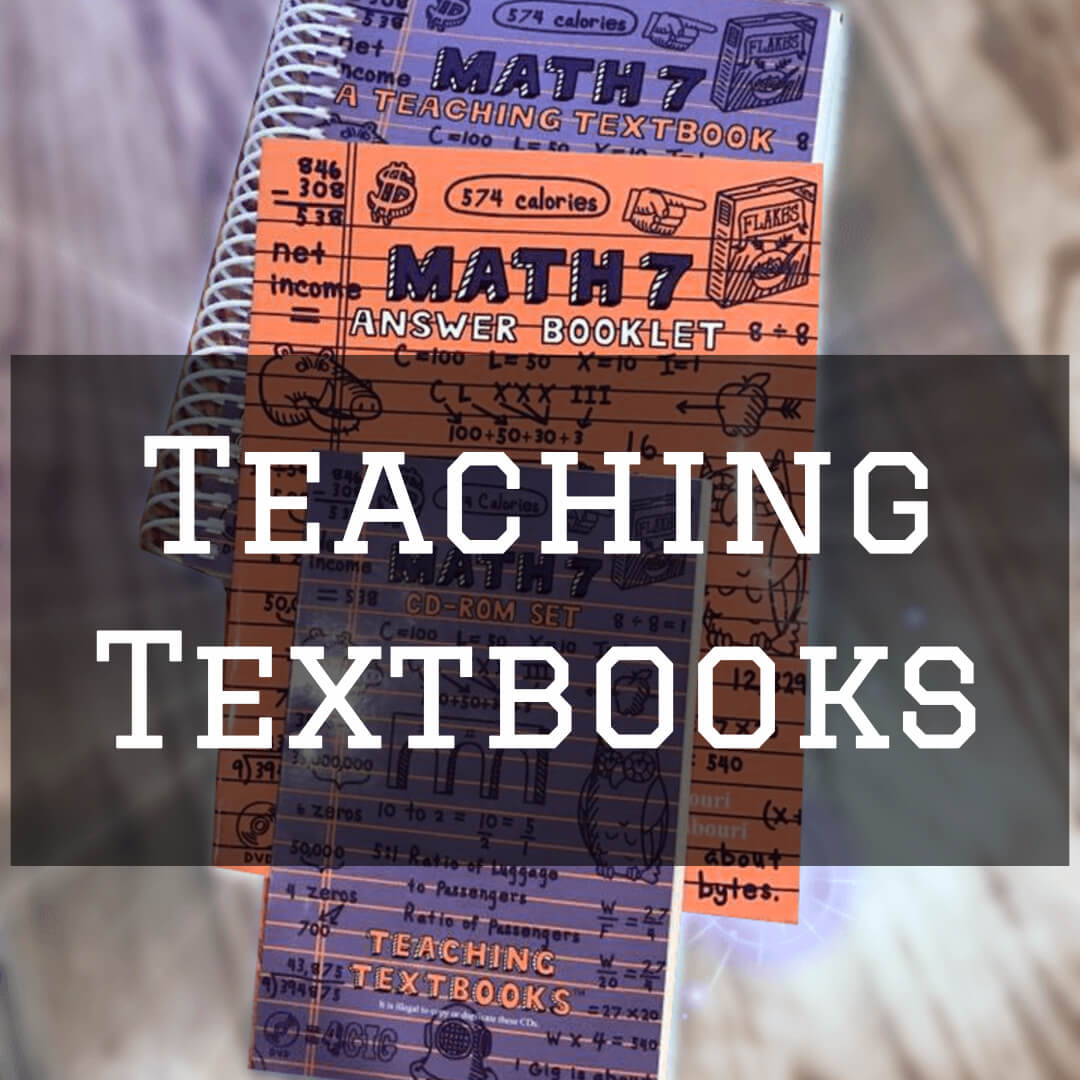## Teaching Textbooks

Teaching Textbooks were specifically designed to solve the issues that make math challenging for homeschoolers. Lessons are taught via CDs that provide audio presentations and step-by-step written exp...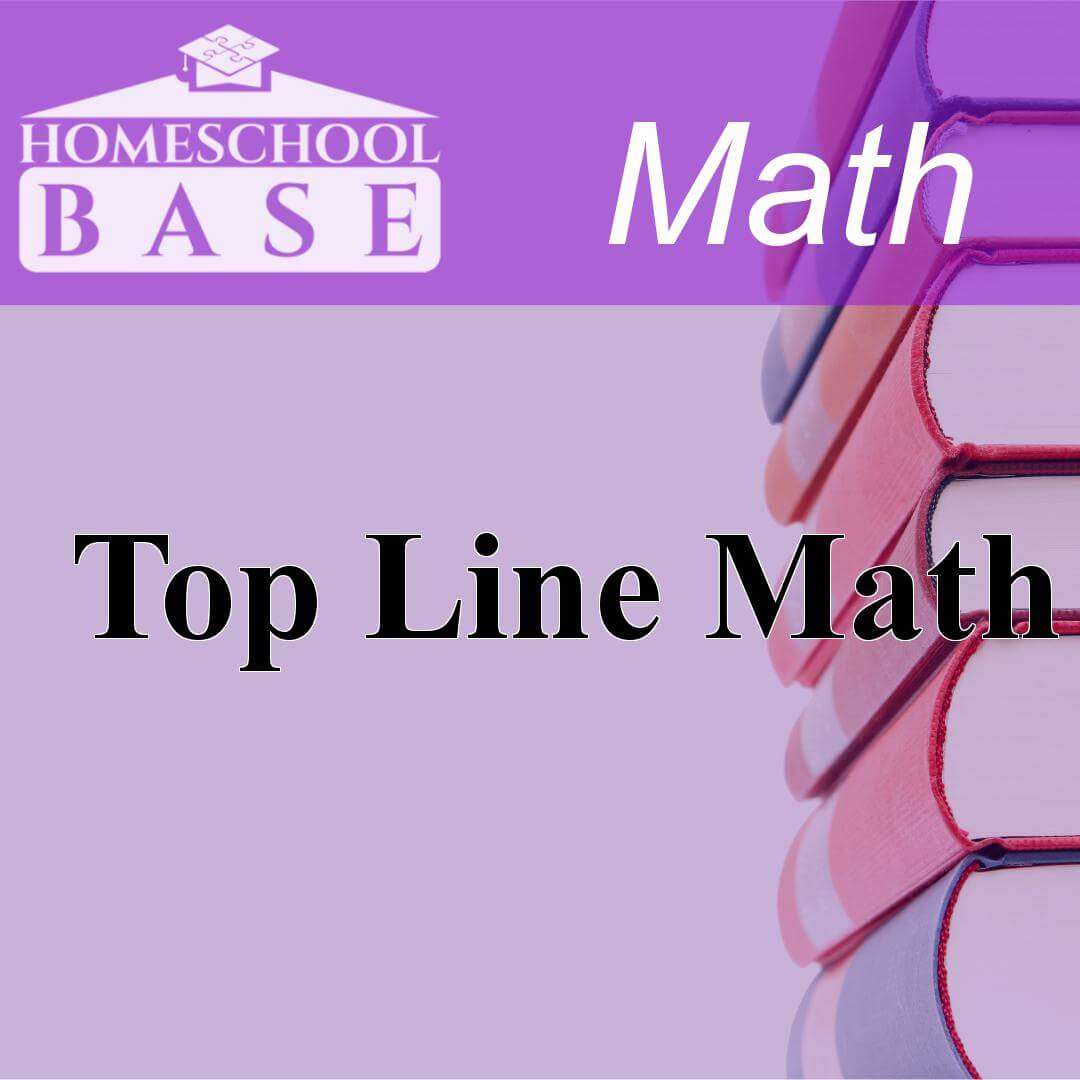## Top Line Math